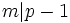# Every finite group admits a sufficiently large finite prime field

## Definition

For any finite group, there exists a prime field (not of characteristic zero) that is sufficiently large with respect to the finite group.

## Definitions used

### Sufficiently large field

Further information: sufficiently large field

A field$k$ is termed sufficiently large with respect to a finite group$G$ if the following are true:

• The characteristic of$k$ does not divide the order of$G$.
•$k$ contains$d$ distinct$d^{th}$ roots of unity, where$d$ is the exponent of$G$. In other words, the polynomial$x^d - 1$ splits completely into linear factors over$k$.

Since the multiplicative group of a prime field is cyclic, a prime field with$p$ elements is sufficiently large with respect to the finite group$G$ iff the exponent of$G$ divides$p - 1$. Similarly, since the multiplicative group of a finite field is cyclic, a finite field of order$q = p^r$ is sufficiently large with respect to the finite group$G$ iff the exponent of$G$ divides$q - 1$.

## Facts used

1. There are infinitely many primes that are one modulo any modulus: This is the easy case of Dirichlet's theorem on primes in arithmetic progressions, which states that given any positive integer$m$, there exist infinitely many primes$p$ such that$m|p-1$.

## Proof

By the definition of sufficiently large, it suffices to find a prime$p$ such that$p$ is congruent to$1$ modulo the exponent of the group. The existence of such a prime is guaranteed by fact (1).